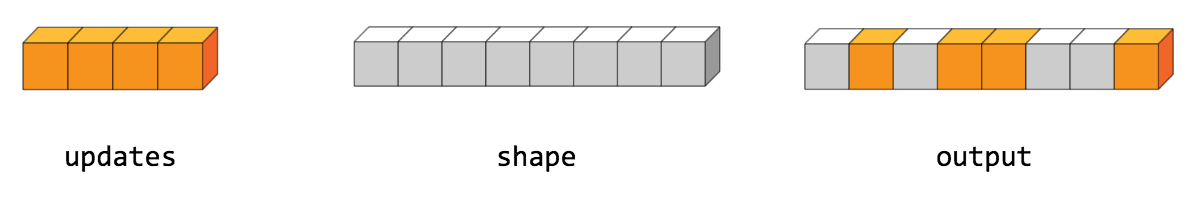Help protect the Great Barrier Reef with TensorFlow on Kaggle

tf.tensor_scatter_nd_update

Scatter updates into an existing tensor according to indices.

This operation creates a new tensor by applying sparse updates to the passed in tensor. This operation is very similar to tf.scatter_nd, except that the updates are scattered onto an existing tensor (as opposed to a zero-tensor). If the memory for the existing tensor cannot be re-used, a copy is made and updated.

If indices contains duplicates, then their updates are accumulated (summed).

indices is an integer tensor containing indices into a new tensor of shape shape. The last dimension of indices can be at most the rank of shape:

indices.shape[-1] <= shape.rank

The last dimension of indices corresponds to indices into elements (if indices.shape[-1] = shape.rank) or slices (if indices.shape[-1] < shape.rank) along dimension indices.shape[-1] of shape. updates is a tensor with shape

indices.shape[:-1] + shape[indices.shape[-1]:]

The simplest form of scatter is to insert individual elements in a tensor by index. For example, say we want to insert 4 scattered elements in a rank-1 tensor with 8 elements.In Python, this scatter operation would look like this:

indices = tf.constant([, , , ])
updates = tf.constant([9, 10, 11, 12])
tensor = tf.ones(, dtype=tf.int32)
with tf.Session() as sess:
print(sess.run(scatter))

The resulting tensor would look like this:

[1, 11, 1, 10, 9, 1, 1, 12]

We can also, insert entire slices of a higher rank tensor all at once. For example, if we wanted to insert two slices in the first dimension of a rank-3 tensor with two matrices of new values.

In Python, this scatter operation would look like this:

indices = tf.constant([, ])
updates = tf.constant([[[5, 5, 5, 5], [6, 6, 6, 6],
[7, 7, 7, 7], [8, 8, 8, 8]],
[[5, 5, 5, 5], [6, 6, 6, 6],
[7, 7, 7, 7], [8, 8, 8, 8]]])
tensor = tf.ones([4, 4, 4])
with tf.Session() as sess:
print(sess.run(scatter))

The resulting tensor would look like this:

[[[5, 5, 5, 5], [6, 6, 6, 6], [7, 7, 7, 7], [8, 8, 8, 8]],
[[1, 1, 1, 1], [1, 1, 1, 1], [1, 1, 1, 1], [1, 1, 1, 1]],
[[5, 5, 5, 5], [6, 6, 6, 6], [7, 7, 7, 7], [8, 8, 8, 8]],
[[1, 1, 1, 1], [1, 1, 1, 1], [1, 1, 1, 1], [1, 1, 1, 1]]]

Note that on CPU, if an out of bound index is found, an error is returned. On GPU, if an out of bound index is found, the index is ignored.

tensor A Tensor. Tensor to copy/update.
indices A Tensor. Must be one of the following types: int32, int64. Index tensor.
updates A Tensor. Must have the same type as tensor. Updates to scatter into output.
name A name for the operation (optional).

A Tensor. Has the same type as tensor.

[]
[]